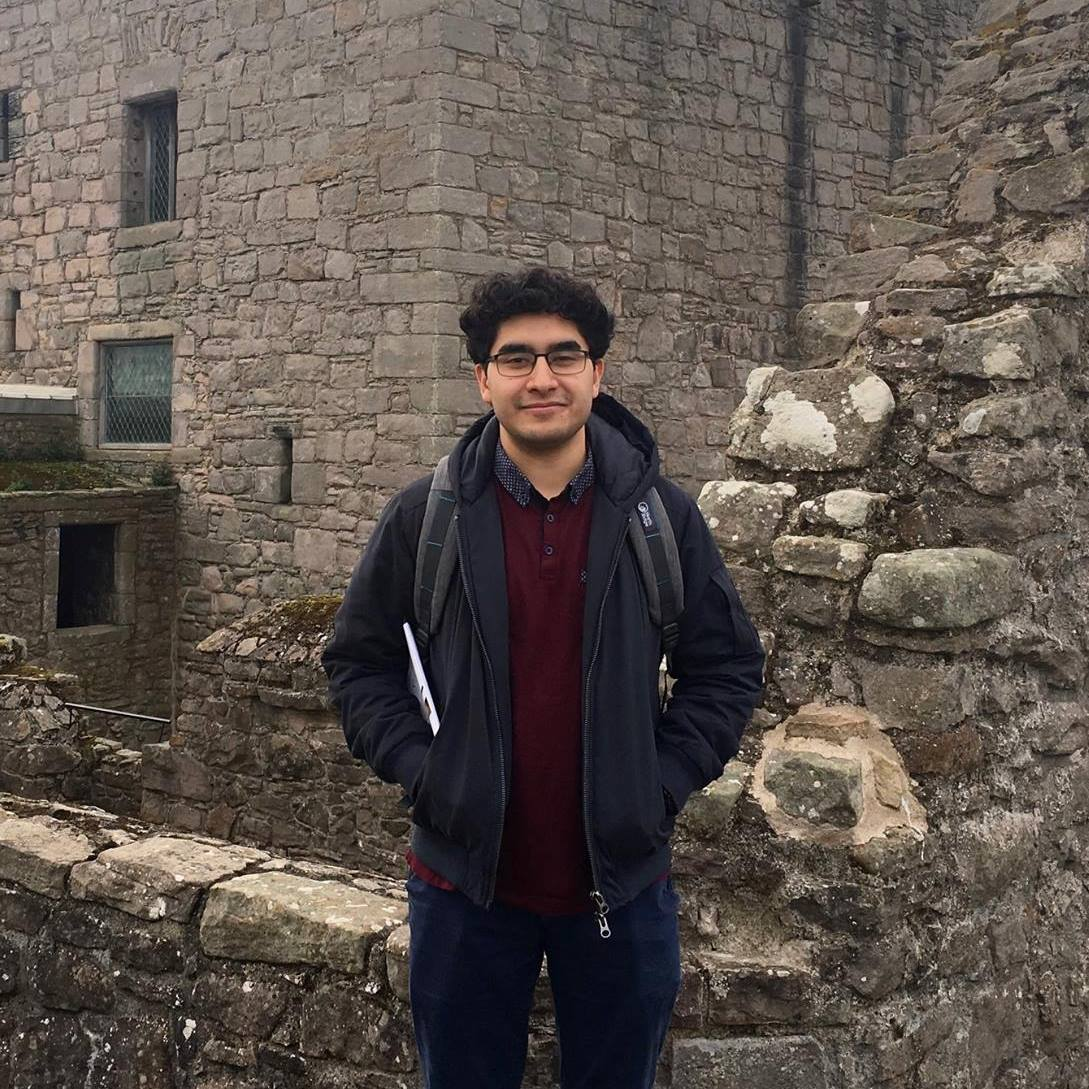• ## Dharmesh TailorDharmesh was a Young Graduate Trainee in Artificial Intelligence from September 2017 to December 2018.

He has a Master’s degree in Artificial Intelligence from the University of Edinburgh, obtained in 2017, in which he specialised in deep learning, reinforcement learning and computational neuroscience. Prior to that, he studied computer science at Imperial College London, graduating in 2016.

### Research

In 2016, the ACT proposed a supervised learning based algorithm for trajectory generation. This novel approach involves caching the solutions of a trajectory optimiser and using the resulting optimal state-control pairs as a dataset to train a machine learning algorithm. Deep neural networks, the state-of-the-art algorithm in machine learning, were used. These models can be trained effectively on large amounts of data and can be easily augmented (e.g. increase the number of layers) to improve the capacity of the model to represent the data. It was also shown that the trained neural network approximates the optimal deterministic policy (i.e. the solution to the Hamilton-Jacobi-Bellman policy equation). Thus we also present this as a method to derive the optimal policy that avoids the curse of dimensionality when directly solving the HJB equations.

In previous work, the ACT demonstrated this approach for a number of landing problems within aerospace including quadcopter (power and time optimal control) and simplified spacecraft models (mass-optimal control). The neural network was able to approximate both continuous and bang-bang control behaviours. We further demonstrated this approach for an Earth-Mars orbital transfer . One of the main advantages of this approach is its suitability for real-time, optimal trajectory generation. The algorithm shows a significant speed-up compared with a trajectory optimiser. This motivates an ongoing study (as of December 2018) with TU Delft which is evaluating the feasibility of this technique for the on-board, optimal control of a quadcopter. Code for this project can be found here.

A significant concern in using a supervised learning based algorithm for trajectory generation is that the resulting controller has no guarantee of stability. For a 2D quadcopter model in which the optimal state-feedback is represented by a neural network, we studied the local stability near an equilibrium point including also with time delays . Across a range of network architectures, we found that there was little correlation between the network’s accuracy at reproducing the optimal trajectories and its stability properties (e.g. critical time delay). Using high-order automatic differentiation (audi), we can represent the state along a nominal trajectory as a Taylor polynomial with respect to deviations from the initial state. With this representation, for small perturbations from the initial state, we analysed convergence properties of the nominal trajectory.

### Publications

1. Izzo D, Sprague C, Tailor D. Machine learning and evolutionary techniques in interplanetary trajectory design. 2018
2. Izzo D, Tailor D, Vasileiou T. On the stability analysis of optimal state feedbacks as represented by deep neural models. 2018